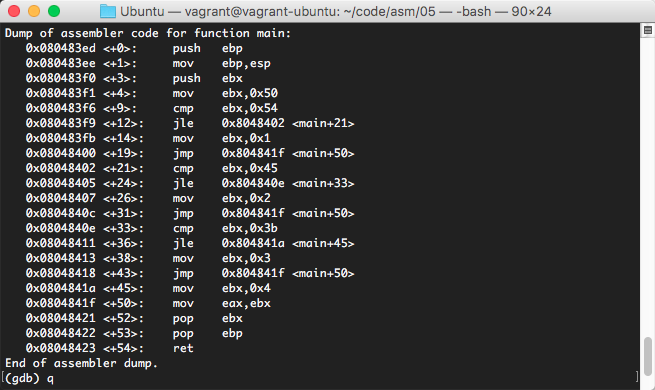• 几条简单的汇编指令
• 寄存器
• 内存访问

• 超级简单的运算
• 变量

顺序执行

``````99+10=
32-20=
14+21=
47-9=
87+3=
86-8=
...``````

CPU中的顺序执行过程

（不要问我为什么，我也不知道，这都是人做出来的东西，支不支持就看人家的心情。反正Intel的CPU做出来就是这个样子的，你可以认为，Intel在做CPU的时候压根就没支持这个功能，他们觉得做了也没什么卵用。虽然你可能觉得有这个功能不是更好么，但是实际上，有时候刻意对功能施加一些限制，可以减少程序员写代码误操作的机会，eip这个东西，很关键）

事情没那么简单

``````if( a < 1 ){
// some code ...
} else if( a >= 10 ) {
// yi xie dai ma ...
}``````

• 更改eip和更改别的寄存器产生的效果不一样，所以应该特殊对待
• 要更改有着特殊用途的eip，就用特殊的指令来完成，虽然都是在更改寄存器，但是代码写出来，表达给人的意思就不一样了

``````global main

main:
mov eax, 1
mov ebx, 2
ret``````

``````eax = 1
ebx = 2
eax = eax + ebx``````

``````global main

main:
mov eax, 1
mov ebx, 2

jmp gun_kai

gun_kai:
ret``````

``````...
jmp gun_kai
...
gun_kai:
...    ``````

``    add eax, ebx``

``````int main() {
int a = 1;
int b = 2;

goto gun_kai;

a = a + b;

gun_kai:
return a;
}``````

if在汇编里的样子

``````int main() {
int a = 50;
if( a > 10 ) {
a = a - 10;
}
return a;
}``````

``````global main

main:
mov eax, 50
cmp eax, 10                         ; 对eax和10进行比较
jle xiaoyu_dengyu_shi            ; 小于或等于的时候跳转
sub eax, 10
xiaoyu_dengyu_shi:
ret``````

``````    cmp eax, 10                         ; 对eax和10进行比较
jle xiaoyu_dengyu_shi            ; 小于或等于的时候跳转``````

• 第一条，cmp指令，专门用来对两个数进行比较
• 第二条，条件跳转指令，当前面的比较结果为“小于或等于”的时候就跳转，否则不跳转

• 我会写a > 10的情况了，那么a < 10怎么办呢？a == 10怎么办呢？a <= 10怎么办呢？a >= 10怎么办呢？

``````if ( a > 10 ) {
a = a - 10;
}``````

``````    cmp eax, 10
jle xiaoyu_dengyu_shi
sub eax, 10
xiaoyu_dengyu_shi:``````

• C语言中：a大于10的时候，进入if块中执行减法
• 汇编语言中：eax小于等于10的时候，跳过中间的减法

C语言和汇编语言中的条件判断，其组织的思路是刚好相反的。这就在编程的时候带来一些思考上的困难，不过这都还是小事情，实在困难你可以先画出流程图，然后对流程图进行改造，就可以了。

``````int main() {
int x = 1;
if ( x > 100 ) {
x = x - 20;
}
x = x + 1;
return x;
}``````

• 对x对应的东西与100进行比较
• 何时跳过if块中的减法
• x = x + 1是无论如何都会执行的

``````global main

main:
mov eax, 1

cmp eax, 100
jle xiao_deng_yu_100
sub eax, 20

xiao_deng_yu_100:
ret``````

``    mov eax, 1``

``    mov eax, 110``

再来套路

• 我会写a > 10的情况了，那么a < 10怎么办呢？a == 10怎么办呢？a <= 10怎么办呢？a >= 10怎么办呢？

• jmp
• jle

• cmp

``````ja 大于时跳转
jae 大于等于
jb 小于
jbe 小于等于
je 相等
jna 不大于
jnae 不大于或者等于
jnb 不小于
jnbe 不小于或等于
jne 不等于
jg 大于(有符号)
jge 大于等于(有符号)
jl 小于(有符号)
jle 小于等于(有符号)
jng 不大于(有符号)
jnge 不大于等于(有符号)
jnl 不小于
jnle 不小于等于
jns 无符号
jnz 非零
js 如果带符号
jz 如果为零``````

What？这该不会都得记住吧？其实不用，这里面是有套路的：

• 首先，跳转指令的前面都是字母j
• 关键是j后面的的字母

• a: above
• e: equal
• b: below
• n: not
• g: greater
• l: lower
• s: signed
• z: zero

``````int main() {
int x = 10;
if ( x > 100 ) {
x = x - 20;
}
if( x <= 10 ) {
x = x + 10;
}
x = x + 1;
return 0;
}``````

``````global main

main:
mov eax, 10

cmp eax, 100
jle lower_or_equal_100
sub eax, 20

lower_or_equal_100:
cmp eax, 10
jg greater_10

greater_10:
ret``````

if都有了，那else if和else怎么办呢？

• 首先根据你的需要，画出整个程序的流程图
• 按照流程图中的跳转关系，通过汇编表达出来

``````int main() {
register int level;
if ( grade >= 85 ){
level = 1;
} else if ( grade >= 70 ) {
level = 2;
} else if ( grade >= 60 ) {
level = 3;
} else {
level = 4;
}
return level;
}``````

（程序中有一个register关键字，是用来限定这个变量在编译后只能用寄存器来进行表示，方便我们进行分析。读者可以根据需要，去掉register关键字后比较一下反汇编代码有何不同。）

``````\$ gcc -m32 grade.c -o grade
2``````

``````\$ gdb ./grade
(gdb) set disassembly-flavor intel
(gdb) disas main``````

``````Dump of assembler code for function main:
0x080483ed < +0>:    push   ebp
0x080483ee < +1>:    mov    ebp,esp
0x080483f0 < +3>:    push   ebx
0x080483f1 < +4>:    mov    ebx,0x50
0x080483f6 < +9>:    cmp    ebx,0x54
0x080483f9 <+12>:    jle    0x8048402 <main+21>
0x080483fb <+14>:    mov    ebx,0x1
0x08048400 <+19>:    jmp    0x804841f <main+50>
0x08048402 <+21>:    cmp    ebx,0x45
0x08048405 <+24>:    jle    0x804840e <main+33>
0x08048407 <+26>:    mov    ebx,0x2
0x0804840c <+31>:    jmp    0x804841f <main+50>
0x0804840e <+33>:    cmp    ebx,0x3b
0x08048411 <+36>:    jle    0x804841a <main+45>
0x08048413 <+38>:    mov    ebx,0x3
0x08048418 <+43>:    jmp    0x804841f <main+50>
0x0804841a <+45>:    mov    ebx,0x4
0x0804841f <+50>:    mov    eax,ebx
0x08048421 <+52>:    pop    ebx
0x08048422 <+53>:    pop    ebp
0x08048423 <+54>:    ret  ``````

• 部分无关指令可以直接忽略掉，如：push、pop等
• 跳转指令后的<main+21>，就对应的是反汇编指令前是<+21>的指令

状态寄存器

• 先比较两个数
• 记住比较结果
• 根据比较结果作出决定

``eflags``

cmp指令实际上是在对两个操作数进行减法，减法后的一些状态最终就会反映到eflags寄存器中。

总结

• eip寄存器指示着CPU接下来要执行哪里的代码
• 一系列跳转指令，跳转指令根本上就是修改了eip
• 比较指令，比较指令实际上是在做减法，然后把结果的一些状态放到eflags寄存器中
• eflags寄存器的作用
• 条件跳转指令也就是根据eflags中的信息来决定是否跳转

• x86标志寄存器
• x86影响标志寄存器的指令
• x86跳转指令